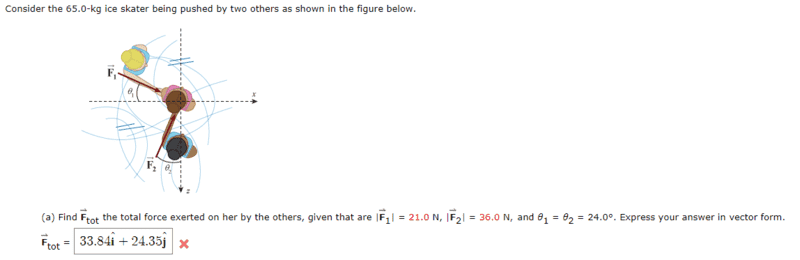# What's the Total Force Exerted on the Skater?

## Homework Statement

"Consider the 65.0-kg ice skater being pushed by two others as shown in the figure below."
Figure: https://gyazo.com/9d7525071f54b00ea7e720abdeb50594Find the total force exerted on her by the others, given that F1 = 21N and F2 = 36N. Express your answer in vectorm form.

## Homework Equations

I doubt "F=ma" is relevant for this question considering we're merely calculating the force directed towards the skater instead of her movement thereafter.

## The Attempt at a Solution

I calculated the components of F1 and F2 with the following numbers:
I judged the angle of F1 to be (360 - 24 = 336).
F1: 21cos336 (x-component) + 21sin336 (y-component).
I judged the angle of F2 to be (90 - 24 = 66).
F2: 36cos66 (x-component) + 36sin66 (y-component).
I calculated the sum of these as 33.84i + 24.35j.
Judging by the picture, are my translations of the angles correct?
Any guidance is appreciated.

#### Attachments

Last edited by a moderator:

RPinPA
Homework Helper
Yes, your analysis of the angles is correct and thus your expressions for the components of ##F_1## and ##F_2## are correct. I haven't checked the numerical calculation but I always consider that to be a small part of the solution.

CWatters
Homework Helper
Gold Member
The axis appear to be labeled X , Z rather than X, Y.

•Austin Gibson
gneill
Mentor
The axis appear to be labeled X , Z rather than X, Y.
Well spotted. And notice the direction of +Z axis•CWatters and Austin Gibson
Aha! That was mildly clumsy of me. I apologize. I appreciate your keen eyes. With this new information, I will redo my calculations. Thank you for your contributions.

•gneill